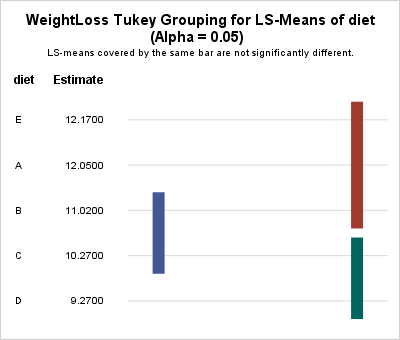Last week Warren Kuhfeld wrote about a graph called the "lines plot" that is produced by SAS/STAT procedures in SAS 9.4M5. (Notice that the "lines plot" has an 's'; it is not a line plot!) The lines plot is produced as part of an analysis that performs multiple comparisons of means. Although the graphical version of the lines plot is new in SAS 9.4M5, the underlying analysis has been available in SAS for decades. If you have an earlier version of SAS, the analysis is presented as a table rather than as a graph.

Warren's focus was on the plot itself, with an emphasis on how to create it. However, the plot is also interesting for the statistical information it provides. This article discusses how to interpret the lines plot in a multiple comparisons of means analysis.

### The lines plot in SAS

You can use the LINES option in the LSMEANS statement to request a lines plot in SAS 9.4M5. The following data are taken from Multiple Comparisons and Multiple Tests (p. 42-53 of the First Edition). Researchers are studying the effectiveness of five weight-loss diets, denoted by A, B, C, D, and E. Ten male subjects are randomly assigned to each method. After a fixed length of time, the weight loss of each subject is recorded, as follows:

```/* Data and programs from _Multiple Comparisons and Multiple Tests_ Westfall, Tobias, Rom, Wolfinger, and Hochberg (1999, First Edition) */ data wloss; do diet = 'A','B','C','D','E'; do i = 1 to 10; input WeightLoss @@; output; end; end; datalines; datalines; 12.4 10.7 11.9 11.0 12.4 12.3 13.0 12.5 11.2 13.1 9.1 11.5 11.3 9.7 13.2 10.7 10.6 11.3 11.1 11.7 8.5 11.6 10.2 10.9 9.0 9.6 9.9 11.3 10.5 11.2 8.7 9.3 8.2 8.3 9.0 9.4 9.2 12.2 8.5 9.9 12.7 13.2 11.8 11.9 12.2 11.2 13.7 11.8 11.5 11.7 ;```

You can use PROC GLM to perform a balanced one-way analysis of variance and use the MEANS or LSMEANS statement to request pairwise comparisons of means among the five diet groups:

```proc glm data=wloss; class diet; model WeightLoss = diet; *means diet / tukey LINES; lsmeans diet / pdiff=all adjust=tukey LINES; quit;```

In general, I use the LSMEANS statement rather than the MEANS statement because LS-means are more versatile and handle unbalanced data. (More about this in a later section.) The PDIFF=ALL option requests an analysis of all pairwise comparisons between the LS-means of weight loss for the different diets. The ADJUST=TUKEY option is a common way to adjust the widths of confidence intervals to accommodate the multiple comparisons. The analysis produces several graphs and tables, including the following lines plot.### How to interpret a lines plot

In the lines plot, the vertical lines visually connect groups whose LS-means are "statistically indistinguishable." Statistically speaking, two means are "statistically indistinguishable" when their pairwise difference is not statistically significant.

If you have k groups, there are k(k-1)/2 pairwise differences that you can examine. The lines plot attempts to summarize those comparisons by connecting groups whose means are statistically indistinguishable. Often there are fewer lines than pairwise comparisons, so the lines plot displays a summary of which groups have similar means.

In the previous analysis, there are five groups, so there are 10 pairwise comparisons of means. The lines plot summarizes the results by using three vertical lines. The leftmost line (blue) indicates that the means of the 'B' and 'C' groups are statistically indistinguishable (they are not significantly different). Similarly, the upper right vertical bar (red) indicates that the means of the pairs ('E','A'), ('E','B'), and ('A','B') are not significantly different from each other. Lastly, the lower right vertical bar (green) indicates that the means for groups 'C' and 'D' are not significantly different. Thus in total, the lines plot indicates that five pairs of means are not significantly different.

The remaining pairs of mean differences (for example, 'E' and 'D') are significantly different. By using only three vertical lines, the lines plot visually associates pairs of means that are essentially the same. Those pairs that are not connected by a vertical line are significantly different.

### Advantages and limitations of the lines plot

Advantages of the lines plot include the following:

• The groups are ordered according to the observed means of the groups.
• The number of vertical lines is often much smaller than the number of pairwise comparisons between groups.

Notice that the groups in this example are the same size (10 subjects). When the group sizes are equal (the so-called "balanced ANOVA" case), the lines plot can always correctly represent the relationships between the group means. However, that is not always true for unbalanced data. Westfall et al. (1999, p. 69) provide an example in which using the LINES option on the MEANS statement produces a misleading plot.

The situation is less severe when you use the LSMEANS statement, but for unbalanced data it is sometimes impossible for the lines plot to accurately connect all groups that have insignificant mean differences. In those cases, SAS appends a footnote to the plot that alerts you to the situation and lists the additional significances not represented by the plot.

In my next blog post, I will show some alternative graphical displays that are appropriate for multiple comparisons of means for unbalanced groups.

### Summary

In summary, the new lines plot in SAS/STAT software is a graphical version of an analysis that has been in SAS for decades. You can create the plot by using the LINES option in the LSMEANS statement. The lines plot indicates which groups have mean differences that are not significant. For balanced data (or nearly balanced), it does a good job of summarizes which differences of means are not significant. For highly unbalanced data, there are other graphs that you can use. Those graphs will be discussed in a future article.

The post Graphs for multiple comparisons of means: The lines plot appeared first on The DO Loop.